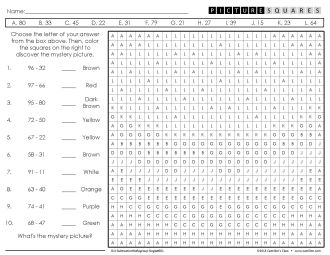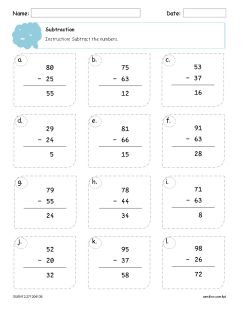This is a collection of our products on subtraction worksheets for 2nd grade. You'll find these resources helpful as it saves you precious time creating your own teaching materials.

Here are some sample images of our products on subtraction.Subtraction Worksheets for Grade 2 - Coloring PageSubtraction Worksheets for 2nd Grade QuestionnaireSubtraction Worksheets for 2nd Grade - Answer Key

Browse our selection of resources. You'll see various activities for your classroom, including classic worksheets, task cards, coloring worksheets and other printables.

Choose the perfect material for your class. Just click on the images or the links below.

### Save it on Pinterest

Click the image on the right to pin this page. You can also share to your friends by click on the buttons on the left.

Here are all the products in this 2nd grade subtraction worksheets collection. You can get more from the selection below. Just choose and click the ones that suits your needs.

##### 2nd Grade Subtraction Without Regrouping Mystery Pictures Coloring Worksheets

There are two versions in this packet. Choose the version that matches your preference. Version 1: Two Pages. Separate questionnaire and coloring pageVersion 2: Single Page. Questions and coloring page are in a single page. Each version is divided into sets. A set has a questionnaire, a coloring page, and an answer...

##### Subtraction Worksheets

This is a collection of all our subtraction worksheets. Save tons of time using this packet. It's great for homework, math centers, and sub-plans, and general practice.  What You'll Get You'll receive several printable files. These are 2-page files with answer keys. They are organized into folders for easy access. Just pick...

##### Double Digit Subtraction Worksheets

This is a collection of double digit subtraction worksheets. These are great for homework, math centers, and sub-plans, and general practice.  Take note that this is a subset of our subtraction worksheets packet. What You'll Get You will receive several printable files. These are 2-page files with worksheets and answer keys. They...

##### 2nd Grade Subtraction Word Problems Mystery Pictures Coloring Worksheets

You will receive a printable file. It is divided into sets. Each set has a questionnaire, coloring page, and an answer key. The sets included in this product are: Sets 1-3: Subtraction word problems (within 100)Sets 4-5: Subtraction word problems (within 1,000) If your kids answered the word problems correctly and...

##### 2nd Grade Two Step Word Problems Mystery Pictures Coloring Worksheets

If you need extra motivation for your students to practice two-step word problems, let them work on these mystery pictures activity. These are coloring worksheets for 2nd grade on addition and subtraction within 100, involving situations of adding to or subtracting from. This is no-prep packet -- just print and you're...

##### 2nd Grade Length Word Problems Mystery Pictures Coloring Worksheets

Let your 2nd graders have fun answering length word problems as they solve the mystery pictures in these coloring activity sheets. This is a no-prep packet -- just print and you're ready to go! To use, hand over the question sheets to your students. You may choose to give your students the coloring...

##### 2nd Grade Subtraction With Regrouping Mystery Pictures Coloring Worksheets

There are two versions of this product. Choose the version that matches your preference. Version 1: Two Page. Separate questionnaire and coloring pageVersion 2: Single Page. Questions and coloring page are in a single page. Each product version is divided into sets, with each set having a questionnaire, a coloring page, and...

This is a bundle of our addition and subtraction worksheets. You will save tons of time with this bundle. These are great for homework, math centers, and sub-plans, and general practice.  Addition Worksheets Subtraction Worksheets What You'll Get You'll receive several printable files. These are 2-page files with answer keys. They are organized...

##### Christmas Subtraction Coloring Worksheets

Give your students a fun activity this Christmas with these mystery picture coloring pages. These worksheets include problems to help them practice subtracting numbers. How to Use This is a no-prep packet. All you have to do is print the coloring worksheets, and you're ready to go.  To solve the...

##### Earth Day Subtraction Coloring Worksheets

You will receive all printable worksheets. There are three versions of this packet, each with a different set of subtraction problems. Subtraction of 2-digit and 1-digit numbers Subtraction of two 2-digit numbers Subtraction of two 3-digit numbers Each version includes seven images. Just print the right type of problem that you want your students...

##### Easter Subtraction Coloring Worksheets

You won't need much time to prepare this Easter themed subtraction activity. All you have to do is choose the right type of problem, print the worksheets, and you're ready to go.  To solve the mystery picture, your students must answer the subtraction problems first. Then, they choose the letter of...

##### End of the Year Subtraction Coloring Worksheets

Finish the school year with a fun activity for your students. Let them review subtraction while enjoying solving the mystery pictures using these coloring worksheets. How to Use To solve the hidden image, your students need to answer all subtraction problems correctly. Then, they have to choose the letter of their answers...

##### Fall/Autumn Subtraction Coloring Worksheets

You will receive all printable subtraction worksheets. There are three versions of this packet, each with a different set of problems. Choose and print the right type of problem that you want your students to work on. Subtraction of 2-digit and 1-digit numbers Subtraction of two 2-digit numbers Subtraction of two 3-digit numbers The...

##### Halloween Subtraction Mystery Pictures Coloring Worksheets

To solve the mystery picture, your students need to answer all subtraction problems correctly. Then, they have to choose the letter of their answers from a list and color the squares to see the hidden picture. Preparing this activity is easy. Just choose and print the right set of problems for...

##### Spring Subtraction Mystery Pictures Coloring Worksheets

Here is a fun activity that your students will enjoy this spring. These are subtraction coloring worksheets that your kids will love to answer in order to solve the mystery picture.  How to Use First, your students need to answer all subtraction problems correctly. After that, they have to choose the...

##### St. Patrick's Day Subtraction Mystery Pictures Coloring Worksheets

You will receive all the printable worksheets. There are three versions of this packet, each with a different set of subtraction problems. Print the type of problem that is right for your kids.  Subtracting 2-digit and 1-digit numbers Subtracting two 2-digit numbers Subtracting two 3-digit numbers The types of problems included...